# Square and Square Roots 8th CBSE

## Square and Square Roots 8th CBSE

Square of a number. Square and Square Roots 8th CBSE

If a natural number m can be expressed as n2 (where n is a natural number), then m is the square root or perfect square.
i.e. if m = n2          (m, n – natural numbers)
E.g. 81 = 3 × 3 × 3 × 3
= 32 × 32 = (3 × 3)2 = 92
Hence, 9 is the square root of 81.
4.2 Properties of Square Root.
Below is the table that has squares of numbers from 1 to 10.If we see the above results carefully, we can conclude that numbers ending with 0, 1, 4, 5, 6, or 9 at units place are perfect squares None of these end with 2, 3, 7 or 8, So numbers that end with 2, 3, 7, 8 are not perfect squares.
Thus, numbers like 122, 457, 183, 928 are not perfect squares.
4.3 One’s digit in square of a number.
(1). The ones digit in the square of number can be determined if the ones digit of the number is known.(2). The number of zeros at the end of a perfect square is always even and double the number of zeros at the end of the number
E.g.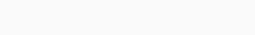Double zero {7002 = 490000} four zero (even)
(3). The square of an even number is always an even number and square of an odd number is always an odd number.
E.g.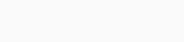4.4 Interesting patterns of Square Root.

Square and Square Roots 8th CBSE

Number between square numbers
There are ‘2a’ non perfect square numbers between the square of two Consecutive natural numbers n + (n + 1)
Between 22 = 4 & 32 = 9 → 5, 6, 7, 8
2 × 2 = 4 non square numbers
Between
32 = 9 & 42 = 16 → 10, 11, 12, 13, 14, 15
2 × 3 = 6 non square numbers.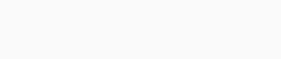So, we can conclude that the sum of first in odd natural numbers in n2 or, we can say if the number is a square number, it has to be the sum of successive odd numbers.
E.g. 36
Successively substract 1, 3, 5, 7 … from 36
36 – 1 = 35
35 – 3 = 32
32 – 5 = 27
27 – 7 = 20
20 – 9 = 11
11 – 11 = 0
∴ 36 is a perfect square
4.5 Short Cut Method of Squaring a number:
We can use the below to methods for Calculating square of any natural number
(a + b)2 = a2 + b2 + 2ab
(a � b)2 = a2 + b2 � 2ab
The logic behind using these formulae is to convert square of unknow number into square of a known number.
E.g.
(53)2 = (50 + 3)2
= 502 + 32 + 2.50.3
= 250 + 9 + 300
= 2809
992 = (100 – 1)2
= 1002 + 12 – 2.100.1
= 10000 + 1 – 200
= 9801
4.6 Square Roots
Finding the Square root is inverse operation of squaring.
If 9 × 9 = 81 i.e, 92 = 81
Then the square root of 81 = 9
In other words, if p = q2, the q is called the square root of p
The Square root of a number is the number which when multiplied with itself gives the number as the product. The square root of a number is denoted by the symbol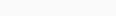Some Properties of square root
1.   The Square root of an even perfect square is even and that of an odd Perfect square is odd.
2.   Since there is no number whose square is negative the square root of a negative number is not defined.
3.   If a number ends with an odd number of zeroes, then it cannot have a square root which is a natural number.
4.   If the units digit of a number is 2, 3, 7 or 8 then square root of that number (in natural numbers) is not possible.
5.   If m is not a perfect square, then there is no integer n such that square root of m is n.
Finding square root through repeated subtraction Recall the pattern formed while adding Consecutive odd numbers.
1 + 3 + 5 + 7 + 9 = 52 = 25
1 + 3 + 5 + 7 + 9 + 11 = 62 = 36
Sum of first n odd numbers = n2
The above Pattern can be used to find the square root of the given number.
1.   Obtain the given perfect square whose square root is to the calculated let the number be a.
2.   Subtract from it successively 1, 3, 5, 7, 9 till you get zero.
3.   Count the number of times the subtraction is performed to arrive at zero let the number be n.
4.   Write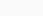Finding square root through Prime factorization. In order to find the square root of a perfect square by prime factorization.
1.   Obtain the given number
2.   Resolve the given number into prime factors by successive division.
3.   Make pairs of prime factors such that both the factors in each pair are equal.
4.   Take one factor from each pair and find their product.
5.   The product obtained is the required square root.
E.g.
Find the square root of 400Finding the square root by division Method when the numbers are large even the method of finding square root by prime factorisation becomes slightly difficult. To over come this problem we use long division method.
Square and Square Roots 8th CBSE
(1).   Consider the following steps to find the square root of 784
Step 1: Place a bar over every pair of digits starting from the digit at one�s place. If the number of digits is odd, then the left most single digit too will have a bar. Thus we have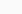Step 2: Find the largest number whose square is less than or equal to the number under the extreme left bar (22 < 7 < 32). Take this number as the divisor and the quotient with the number under the extreme left bar as the dividend (here 7) Divide and get the remainder (5 in this case)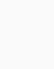Step 3: Bring down the number under the next bar (i.e, 84 in this case) to the right of the remainder. So the new divided is 384.Step 4: Double the divisor and enter it with a blank on its rightStep 5: Gives a largest possible digit to fill the blank which will also become the new digit in the quotient, such that when the new divisor is multiplied to the new quotient the product is less than or equal to the dividend.In this case 47 × 7 = 329
As 48 × 8 = 384 so we choose
the new digit as 8. Get the remainder
Step 6: Since the remainder is 0 and no digits are left in the given number, therefore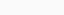Square and Square Roots 8th CBSE
EXERCISE 6.1
1. What will be the unit digit of the squares of the following numbers?
(i) 81(ii) 272(iii) 799(iv) 3853 (v) 1234(vi) 26387(vii) 52698(viii) 99880 (ix) 12796(x) 55555
Sol: (i) ∵ 1 × 1 = I
∴ The unit’s digit of (81)2 will be 1.
(ii) 2 × 2 = 4
The unit’s digits of (272)2 will be 4.
(iii) Since, 9 × 9 = 81
The unit’s digit of (799)2 will be 1.
(iv) Since, 3 × 3 = 9
The unit’s digit of (3853)2 will be 9.
(v) Since, 4 × 4 = 16
The unit’s digit of (1234)2 will be 6.
(vi) Since 7 × 7 = 49
The unit’s digit of (26387)2 will be 9.
(v) Since, 8 × 8 = 64
The unit’s digit of (52698)2 will be 4.
(vi) Since 0 × 0 = 0
The unit’s digit of (99880)2 will be O.
(vii) Since 6 × 6 = 36
The unit’s digit of (12796)2 will be 6.
(x) Since, 5 × 5 = 25
The unit’s digit of (55555)2 will be 5.
2. The following numbers are obviously not perfect squares. Give reason.
(i) 1057(ii) 23453(iii) 7928(iv) 222222 (v) 64000(vi) 89722(vii) 222000(viii) 505050
Sol: (i) 1057
Since, the ending digit is 7 (which is not one of 0, 1, 4, 5, 6 or 9)
∴1057 is not a perfect square.
(ii) 23453
Since, the ending digit is 7 (which is not one of 0, 1, 4, 5, 6 or 9).
∴23453 is not a perfect square.
(iii) 7928
Since, the ending digit is 8 (which is not one of 0, 1, 4, 5, 6 or 9).
∴7928 is not a perfect square.
(iv) 222222
Since, the ending digit is 2 (which is not one of 0, 1, 4, 5, 6 or 9).
∴222222 is not a perfect square.
(v) 64000
Since, the number of zeros is odd.
∴64000 is not a perfect square.
(vi) 89722
Since, the ending digits is 2 (which is not one of 0, 1, 4, 5, 6 or 9).
∴89722 is not a perfect square.
(viii) 222000
Since, the number of zeros is odd.
∴222000 is not a perfect square.
(viii) 505050
The unit’s digit is odd zero.
∴505050 can not be a perfect square.
3. The squares of which of the following would be odd numbers?
(i) 431(ii) 2826(iii) 7779(iv) 82004
Sol: Since the square of an odd natural number is odd and that of an even number is an even number.
(i) The square of 431 is an odd number.
[∵ 431 is an odd number.]
(ii) The square of 2826 is an even number.
[∵ 2826 is an even number.]
(iii) The square of 7779 is an odd number.
[∵ 7779 is an odd number.]
(v) The square of 82004 is an even number.
[∵ 82004 is an even number.]
4. Observe the following pattern and find the missing digits.
112 =
121
1012 =
10201
10012 =
1002001
1000012 =
1………….2 …………. 1
100000012 =
…………
Sol: Observing the above pattern, we have
(i) (100001)2 – 10000200001
(ii) (10000001)2 = 100000020000001
5. Observe the ,following pattern and supply the missing number.
112 = 121
1012 = 10201
101012 = 102030201
10101012 =
…………..2 = 10203040504030201
Sol: Observing the above, we have
(i) (1010101)2 = 1020304030201
(ii) 10203040504030201 = (101010101)2
6. Using the given pattern, find the missing numbers.
12 + 22 + 22 = 32
22 + 32 + 62 = 72
32 + 42 + 122 =132
42 + 52 + —2 = 212
52 + —2 + 302 = 312
62 + 72 + —2 = —2
Note: To find pattern:
Third number is related to first and second number. How?
Fourth number is related to third number. How?
Sol: The missing numbers are
(i) 42 + 52 + 202 = 212
(ii) 52 + 22 + 302 = 312
(iii) 62 + 72 + 422 = 432
7. Without adding, find the sum.
(i) 1 + 3 + 5 + 7 + 9
(ii) 1 + 3 + 5 + 7 + 9 + 11 + 13 + 15 + 17 + 19
(iii) 1 + 3 + 5 + 7 + 9 + 11 + 13 + 15 + 17 +19
Sol: (i) The sum of first 5 odd = 52
= 25
(ii) The sum of first 10 odd numbers = 102
= 100
(iii) The sum of first 12 odd numbers = 122
= 144
8. (i) Lxpress 49 as the sum of 7 odd numbers.
(ii) Express 121 as the sum of 11 odd numbers.
Sol: (i) 49 = 72 = Sum of first 7 odd numbers
= 1 + 3 + 5 + 7 + 9 + 11 + 13
(ii) 121 = 112 = Sum of first 11 odd numbers
= 1 + 3 + 5 + 7 + 9 + 11 + 13 + 15 + 17 + 19 + 21
9. How many numbers lie between squares of the following numbers?
(i) 12 and 13(ii) 25 and 26(iii) 99 and 100
Sol: Since between n2 and (n + 1)2, there are 2n non-square numbers.
∴ (i) Between 122 and 132, there are 2 × 12, i.e. 24 numbers
(ii) Between 252 and 262, there are 2 × 25, i.e. 50 numbers
(iii) Between 992 and 1002, there are 2 × 99, i.e. 198 numbers
EXERCISE 6.2 Square and Square Roots 8th CBSE
1. Find the square of the following numbers.
(i) 32(ii) 35(iii) 86 (iv) 93(v) 71(vi) 46
Sol: (i) (32)2 = (30 + 2)2
= 302 + 2(30)(2) + (2)2
= 900 + 120 + 4 = 1024
(ii) (35)2 = (30 + 5)2
= (30)2 + 2(30)(5) + (5)2
= 900 + 300 + 25
= 1200 + 25 = 1225
Second method
352 = 3 × (3 + 1) × 100 + 25
= 3 × 4 × 100 + 25
= 1200 + 25 = 1225
(iii) (86)2 = (80 + 6)2
= (80)2 + 2(80)(6) + (6)2
= 6400 + 960 + 36 = 7396
(iv) (93)2 = (90 + 3)2
= (90)2 + 2(90)(3) + (3)2
= 8100 + 540 + 9 – 8649
(v) (71)2 = (70 + 1)2
= (70)2 + 2(70)(1) + (1)2
= 4900 + 140 + 1 = 5041
(vi) (46)2 = (40 + 6)2
= (40)2 + 2(40)(6) + (6)2
= 1600 + 480 + 36 = 2116
2. Write a Pythagorean triplet whose one member is
(i) 6(ii) 14(iii) 16(iv) 18
Sol: (i) Let 2n =6 ∴n=3
Now, n2 – 1 = 32 –1 = 8
and n2 + 1 = 32 + 1 = 10
Thus, the required Pythagorean triplet is 6, 8, 10.
(ii) Let 2n = 14 ∴n = 7
Now, n2 – 1 = 72 – 1 = 48
and n2 + 1 = 72 + 1 = 50
Thus, the required Pythagorean triplet is 14, 48, 50.
(iii) Let 2n = 16 /n = 8
Now, n2 – 1 = 82 – 1
= 64 – 1 = 63
and n2 + 1 = 82 + 1
=64 + 1 = 65
∴ The required Pythagorean triplet is 16, 63, 65.
(iv) Let 2n = 18 n = 9
Now, n2 – 1 = 92 – 1
=81 – 1 = 80
and n2 + 1 = 92 + 1
= 81 + 1 = 82
∴ The required Pythagorean triple is 18, 80, 82.
EXERCISE 6.3
1. What could be the possible ‘one’s digits of the square root of each of the following numbers?
(i) 9801(ii) 99856(iii) 998001(iv) 657666025

Sol: The possible digit at one’s place of the square root of:
(i) 9801 can be 1 or 9.
[∵ 1 × 1 = 1 and 9 × 9 = 81]

(ii) 99856 can be 4 or 6.
[∵ 4 × 4 = 16 and 6 × 6 = 36]

(iii) 998001 can be 1 or 9.

(iv) 657666025 can be 5. [∵ 5 × 5 = 25]

2. Without doing any calculation, find the numbers which are surely not /)er/’c’t squares.
(i) 152(ii) 257(iii) 408(iv) 441

Sol: We know that the ending digit of perfect square is 0, 1, 4, 5, 6, and 9.
∴ A number ending in 2, 3, 7 or 8 can never be a perfect square.
(i) 153, cannot be a perfect square.
(ii) 257, cannot be a perfect square.
(iii) 408, cannot be a perfect square.
(iv) 441, can he a perfect square.
Thus, (1) 153, (ii) 257 and (iii) 408 are surely not perfect squares.
3. Find the square roots of. 100 and 169 by the method of repeated subtraction.

4. Find the square roots of the following numbers by the Prime Factorisation Method.
(i) 729(ii) 400(iii) 1764(iv) 4096 (v) 7744(vi) 9604(vii) 5929(viii) 9216 (ix) 529(x) 8100
Square and Square Roots 8th CBSE